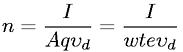Equations > Physics > Electricity and Magnetism > Number density of charge carriers

### Number density of charge carriersLatex Code:

MathML Code:

 $n=\fracI\mathrm{Aq}{\upsilon }_{d}=\fracI\mathrm{wte}{\upsilon }_{d}$

MathType 5.0: